×

Search anything:

# Syntax-Directed Translation Schemes

#### Compiler DesignGet this book -> Problems on Array: For Interviews and Competitive Programming

1. Introduction.
2. Postfix translation schemes.
3. Parser-stack implementation of postfix SDTs.
4. SDTs with actions inside productions.
5. Eliminating left-recursion.
6. SDTs for L-Attributed definitions.
7. Summary.
8. References.

## Introduction.

A translation scheme is a context-free grammar whereby semantic rules are embedded within the right sides of productions.

A translation schema and a syntax-directed definition are close to being similar except that the order for evaluation of semantic rules is shown.

When we construct a parse tree for a translation scheme, we use an extra child that is connected by a dashed line to the node of the production to indicate an action.

In the parse tree, the order in which actions appear is the order in which they are executed.

## Postfix translation schemes.

These are SDTs that have all their actions at the right ends of the production body.
The following is an example of a postfix SDT implementation of a calculator;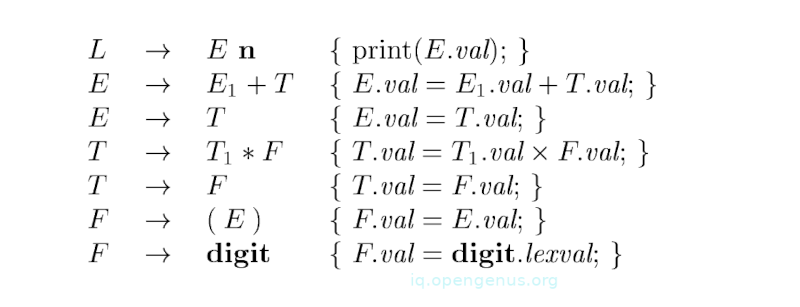Since the underlying grammar is LR and the SDD is S-attributed, actions can be correctly performed along with reduction steps of the parser.

## Parser-Stack implementation of postfix SDTs.

Postfix SDTs can be implemented during LR parsing by executing actions when reductions occur. Attributes of each grammar symbol can be placed on the stack in a place they can be found during reduction. The best way is to place attributes along with grammar symbols in records on the stack itself.

The following is a parser that contains records with a field for a grammar symbol and below it a field for an attribute.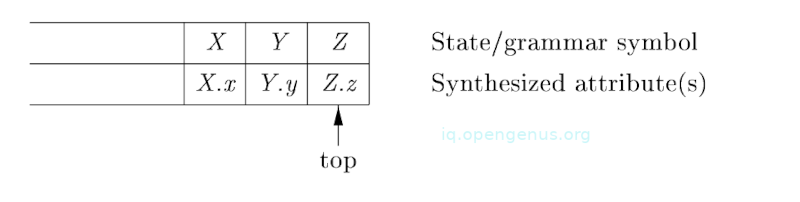The grammar symbols XYZ are to be reduced according to production A $\to$ XYZ.

The desk calculator in a bottom-up parsing stack;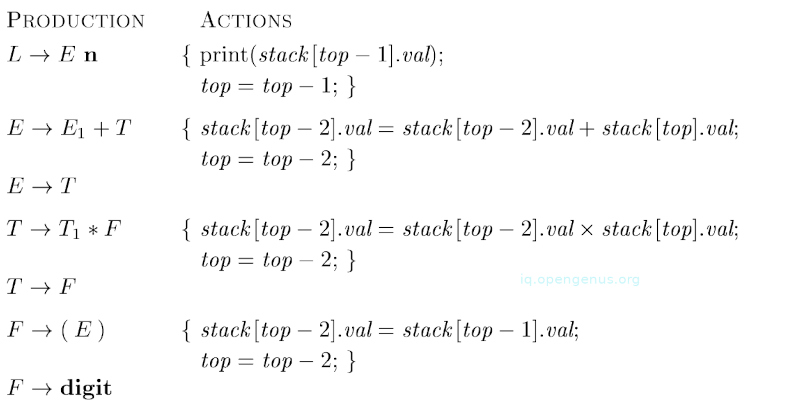## SDTs with actions inside productions.

Take for example a production B $\to$ X{a} Y. Action a is performed after we recognize X(X is a terminal) or all terminals derived from X(X is a non-terminal)

We insert marker non-terminals so as to remove embedded actions and change the SDT into a postfix SDT then rewrite the product with marker non-terminal M into B $\to$ XMY
M $\to$ $\epsilon$ {a}.

Note that inserting marker non-terminals may introduce conflicts in the parse table.

Any SDT can be implemented as listed;

1. First we ignore actions, parse the input and return a parse tree as the result.
2. We then examine each interior node N, for a production A $\epsilon$ $\alpha$. Add more children to N for the actions in $\alpha$, therefore the children of N from left to right will have exactly symbols and actions of $\alpha$.
3. Finally we perform a pre-order traversal of the tree and when we visit a node labeled by an action, we perform the action.

The following is a parse tree for an expression 3 * 5 + 4;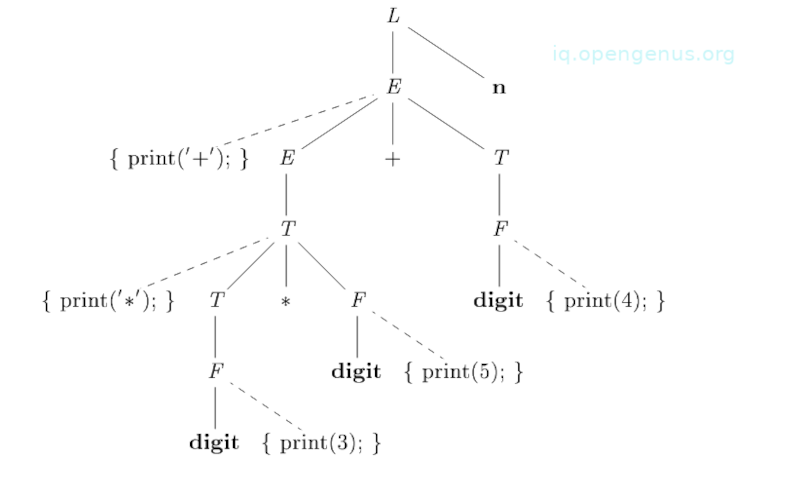## Eliminating left recursion.

No grammar with left-recursion can be parsed deterministically in a top-down manner.
The following principle guides us;

When the order in which actions in an SDT is considered, actions are treated like terminal symbols during grammar transformation.

This principle is based on the idea that grammar transformations preserve the order of the terminals in the generated string.
Actions are therefore executed in any left-to-right, top-down, or bottom-up ordering.

The key to eliminating left recursion is to take two productions;

A $\to$ A$\alpha$ | $\beta$

That generate strings that consist of a $\beta$ and any number of $\alpha$ and replace them by productions that generate a similar string using a new non-terminal R of the first production;

A $\to$ $\beta$R
R $\to$ $\alpha$R | $ϵ$

If $\beta$ does not start with A, A no longer has a left-recursive production.

## SDTs for L-Attributed Definitions.

We assume a grammar can be parsed top-down. Rules for converting an L-Attributed SDD to an SDT are as follows;

1. We embed the action that computes inherited attributed for a non-terminal A that comes immediately before the occurrence of A in the production body. If several inherited attributes for A depend on one another in an acyclic order, the evaluation of those attributes is computed first.
2. Secondly, we place actions that compute a synthesized attribute for the head of a production at the end of the production body.

An example;
Given the grammar;
B $\to$ ${\mathrm{B}}_{1}$${\mathrm{B}}_{2}$ | ${\mathrm{B}}_{1}$ sub ${\mathrm{B}}_{2}$ | (${\mathrm{B}}_{1}$) | text

Although the above grammar is ambiguous we can use it to parse in a bottom-up manner, that is, if we make subscribing and juxtaposition right-associative with the former taking precedence over the latter.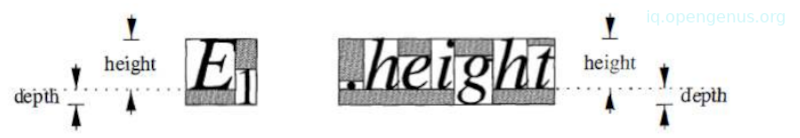The SDD for typesetting boxes;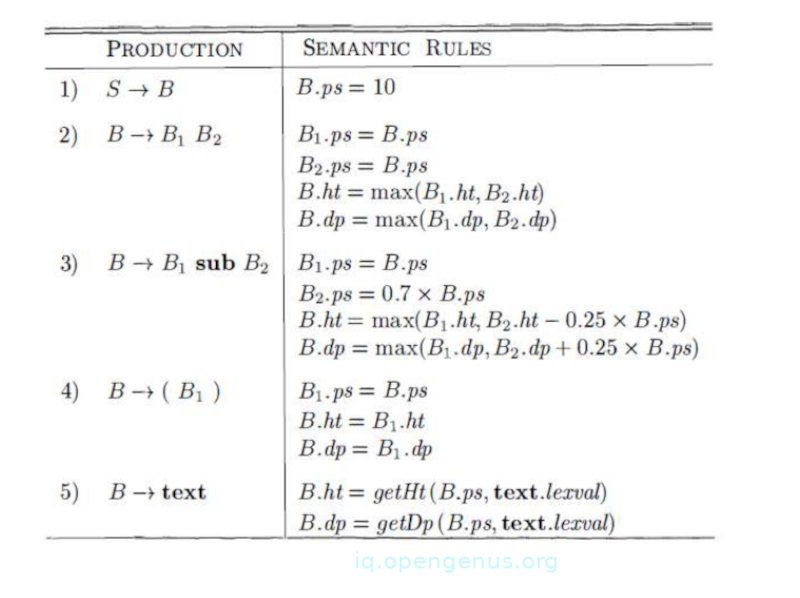The SDT for typesetting boxes;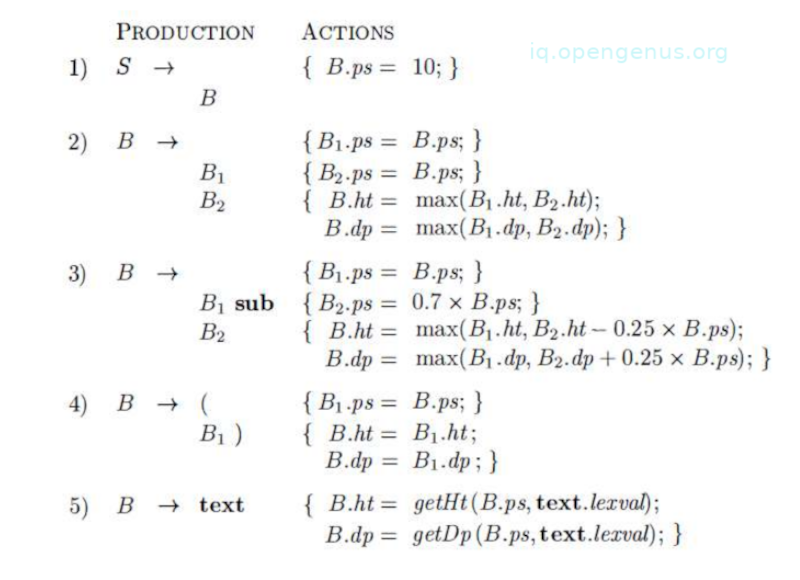## Summary.

A translation scheme is a context-free grammar whereby semantic rules are embedded within the right sides of productions.

In the parse tree, the order in which actions appear is the order in which they are executed.

No grammar with left-recursion can be parsed deterministically in a top-down manner.

Compilers Principles, Techniques, & Tools - Alfred V. Aho, Monica S. Lam

#### Erick Lumunge

Erick is a passionate programmer with a computer science background who loves to learn about and use code to impact lives positively.